Courses

# Test: Line & Bar Charts - 1

## 10 Questions MCQ Test Topic-wise Past Year Questions for CAT | Test: Line & Bar Charts - 1

Description
This mock test of Test: Line & Bar Charts - 1 for CAT helps you for every CAT entrance exam. This contains 10 Multiple Choice Questions for CAT Test: Line & Bar Charts - 1 (mcq) to study with solutions a complete question bank. The solved questions answers in this Test: Line & Bar Charts - 1 quiz give you a good mix of easy questions and tough questions. CAT students definitely take this Test: Line & Bar Charts - 1 exercise for a better result in the exam. You can find other Test: Line & Bar Charts - 1 extra questions, long questions & short questions for CAT on EduRev as well by searching above.
QUESTION: 1

### Directions for Question: Simple Happiness index (SHI) of a country is computed on the basis of three parameters: social support (S), freedom to life choices (F) and corruption perception (C). Each of these three parameters is measured on a scale of 0 to 8 (integers only). A country is then categorised based on the total score obtained by summing the scores of all the three parameters, as shown in the following table:  (2017)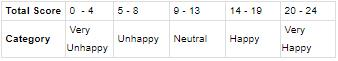Following diagram depicts the frequency distribution of the scores is S, F and C of 10 countries-Amda, Benga, Calla, Delma, Eppa, Varsa, Wanna, Xanda, Yanga and Zooma: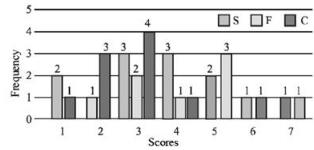Further, the following are known: (a) Amda and Calla jointly have the lowest total score, 7, with identical scores in all the three parameters. (b) Zooma has a total score of 17. (c) All the 3 countries, which are categorised as happy, have the highest score in exactly one parameter. Q. What is Amda's score in F?

Solution:

The given data can be represented in a table as follows.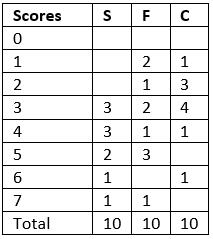A and C had a total score of 7, with identical scores in all these parameters. So it can only be 1, 2 and 4 or 3 and 1.  As Zooma has a score of 17, and all three countries in the happy category had the highest score in exactly one parameter, he can only have a 7 in F, 6 in S and 4 in C as a score of 7 in S and 6 in C would be the scores of the other two countries and he can not have a 7, 7 and 5 as there is no country which scored a 5 in C
Amda can have a distribution of 3, 3, 1 or 4, 2, 1. In either case the only possible score of F is 1 as no other parameter has a scare of 1 for two countries.

QUESTION: 2

### Directions for Question: Simple Happiness index (SHI) of a country is computed on the basis of three parameters: social support (S), freedom to life choices (F) and corruption perception (C). Each of these three parameters is measured on a scale of 0 to 8 (integers only). A country is then categorised based on the total score obtained by summing the scores of all the three parameters, as shown in the following table:  (2017)Following diagram depicts the frequency distribution of the scores is S, F and C of 10 countries-Amda, Benga, Calla, Delma, Eppa, Varsa, Wanna, Xanda, Yanga and Zooma:Further, the following are known: (a) Amda and Calla jointly have the lowest total score, 7, with identical scores in all the three parameters. (b) Zooma has a total score of 17. (c) All the 3 countries, which are categorised as happy, have the highest score in exactly one parameter. Q. What is Zooma's score in S?

Solution:

The given data can be represented in a table as follows.A and C had a total score of 7, with identical scores in all these parameters. So it can only be 1, 2 and 4 or 3 and 1.  As Zooma has a score of 17, and all three countries in the happy category had the highest score in exactly one parameter, he can only have a 7 in F, 6 in S and 4 in C as a score of 7 in S and 6 in C would be the scores of the other two countries and he cannot have a 7, 7 and 5 as there is no country which scored a 5 in C
As explained before Zooma's score in C has to be 6.

QUESTION: 3

### Directions for Question: Simple Happiness index (SHI) of a country is computed on the basis of three parameters: social support (S), freedom to life choices (F) and corruption perception (C). Each of these three parameters is measured on a scale of 0 to 8 (integers only). A country is then categorised based on the total score obtained by summing the scores of all the three parameters, as shown in the following table:  (2017)Following diagram depicts the frequency distribution of the scores is S, F and C of 10 countries-Amda, Benga, Calla, Delma, Eppa, Varsa, Wanna, Xanda, Yanga and Zooma:Further, the following are known: (a) Amda and Calla jointly have the lowest total score, 7, with identical scores in all the three parameters. (b) Zooma has a total score of 17. (c) All the 3 countries, which are categorised as happy, have the highest score in exactly one parameter. Q. Benga and Delma, two countries categorized as happy, are tied with the same total score. What is the maximum score they can have?

Solution:

The given data can be represented in a table as follows.A and C had a total score of 7, with identical scores in all these parameters. So it can only be 1, 2 and 4 or 3 and 1.  As Zooma has a score of 17, and all three countries in the happy category had the highest score in exactly one parameter, he can only have a 7 in F, 6 in S and 4 in C as a score of 7 in S and 6 in C would be the scores of the other two countries and he cannot have a 7, 7 and 5 as there is no country which scored a 5 in C
In the table given, among the highest scores, a score of 7 in F, 6 in S and 4 in S were the score of Zoom. The best possible scores remaining for Benga and Dalma would be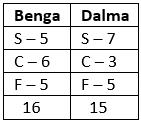As it is given that both had the some total score it can only be 15 for both, i.e. Benga’s score in S or F was one less than the maximum possible.

QUESTION: 4

Directions for Question: Simple Happiness index (SHI) of a country is computed on the basis of three parameters: social support (S), freedom to life choices (F) and corruption perception (C). Each of these three parameters is measured on a scale of 0 to 8 (integers only). A country is then categorised based on the total score obtained by summing the scores of all the three parameters, as shown in the following table:

(2017)Following diagram depicts the frequency distribution of the scores is S, F and C of 10 countries-Amda, Benga, Calla, Delma, Eppa, Varsa, Wanna, Xanda, Yanga and Zooma:Further, the following are known:
(a) Amda and Calla jointly have the lowest total score, 7, with identical scores in all the three parameters.
(b) Zooma has a total score of 17.
(c) All the 3 countries, which are categorised as happy, have the highest score in exactly one parameter.

Q. If Benga scores 16 and Delma scores 15, then what is the maximum number of countries with a score of 13?

Solution:

The given data can be represented in a table as follows.A and C had a total score of 7, with identical scores in all these parameters. So it can only be 1, 2 and 4 or 3 and 1.  As Zooma has a score of 17, and all three countries in the happy category had the highest score in exactly one parameter, he can only have a 7 in F, 6 in S and 4 in C as a score of 7 in S and 6 in C would be the scores of the other two countries and he cannot have a 7, 7 and 5 as there is no country which scored a 5 in C
Considering the score of Zoom, Benga and Delma as 17, 16 and 15, we get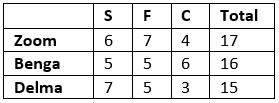If Benga score 16 and Dalma score 15 (as illustrated in the previous solution) the maximum possible values remaining are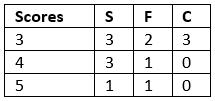QUESTION: 5

Directions for Question: Answer the questions on the basis of the information given below.

Each point in the graph below shows the sales and expenses of a company. Each company belongs to one of the three sectors among manufacturing, automobiles, and software.

(2016)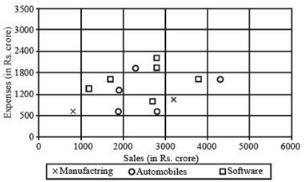Q. For how many of the companies, is the profit more than 40% of the sales (Profit = Sales Expenses)?

Solution:

Companies for which the expenses are less than 60% of the sales, will have a profit more than 40% of the sales. There are six such companies.

QUESTION: 6

Directions for Question: Answer the questions on the basis of the information given below.

Each point in the graph below shows the sales and expenses of a company. Each company belongs to one of the three sectors among manufacturing, automobiles, and software.

(2016)Q. For how many software companies are the sales more than ₹ 2500 crore but the expenses less than ₹ 2100 crore?

Solution:

According to graph, Only three software companies are the sales, more than ₹ 2500 crore and expenses are less than ₹ 2100 crore.

QUESTION: 7

Directions for Question: Answer the questions on the basis of the information given below.
The following pie chart gives the distribution of the total loans disbursed by ADB in 2012 among eleven Asian countries.
Total amount of loans disbursed = ₹ 7200 cr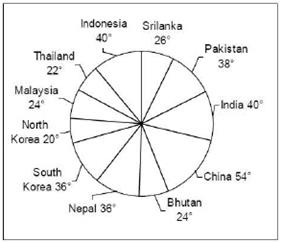The following line graph gives the percentage contribution of loan from ADB in the total investment made in different sectors in the same year by India and China.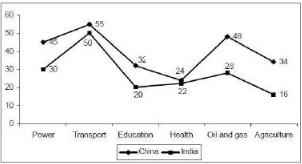For both China and India, the loan received from ADB was utilized in the given sectors only.

(2015)

Q. If the total investment in Education sector in China was 60% higher than that in India, then what is the ratio of A and B, where
A: The percentage of loan from ADB invested in Education sector by China
B: The percentage of loan from ADB invested in Education sector by India

Solution:

Let the total investment in education sector by India = x
Then,
Total investment in education sector by China = 1.6x
According to question, For china. 32% of 1.6x was from ADB loans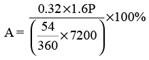For India, 20% of x was from ADB loans.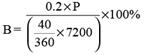Required ratio A : B  = 256 : 135

QUESTION: 8

Directions for Question: Answer the questions on the basis of the information given below.
The following pie chart gives the distribution of the total loans disbursed by ADB in 2012 among eleven Asian countries.
Total amount of loans disbursed = ₹ 7200 crThe following line graph gives the percentage contribution of loan from ADB in the total investment made in different sectors in the same year by India and China.For both China and India, the loan received from ADB was utilized in the given sectors only.

(2015)

Q. The amount of loan invested in Transport sector by China was equal to 60% of the total loan given by ADB to Malaysia. The amount of loan invested in Transport sector by India was equal to 60% of the total loan given by ADB to North Korea. The total investment made in Transport sector by India was approximately what percent of that made by china?

Solution:
QUESTION: 9

Directions for Question: Answer the questions on the basis of the information given below.
The following pie chart gives the distribution of the total loans disbursed by ADB in 2012 among eleven Asian countries.
Total amount of loans disbursed = ₹ 7200 crThe following line graph gives the percentage contribution of loan from ADB in the total investment made in different sectors in the same year by India and China.For both China and India, the loan received from ADB was utilized in the given sectors only.

(2015)

Q. If the total investments made in Education, Health and Agriculture sectors in India in 2012 was ₹ 150 cr., ₹ 120 cr and ₹ 400 cr. respectively, then the amount of ADB loan invested by India in these three sectors constitute what percentage of the total loan granted to India by ADB?

Solution:

Loan amount invested in education = 20% of 150 = ₹ 30 r
Loan amount invested in Health = 22% of 120 = ₹ 26.4 cr
Loan amount invested in Agriculture = 16% of 400 = ₹ 64 cr
∴ Total loan amount invested in India = 40 / 360 x 7200
The required percentage =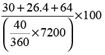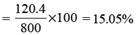QUESTION: 10

Directions for Question: Answer the questions on the basis of the information given below.
The following pie chart gives the distribution of the total loans disbursed by ADB in 2012 among eleven Asian countries.
Total amount of loans disbursed = ₹ 7200 crThe following line graph gives the percentage contribution of loan from ADB in the total investment made in different sectors in the same year by India and China.For both China and India, the loan received from ADB was utilized in the given sectors only.

(2015)

Q. The total loan invested in Power, Transport and Education sectors by India was 500 cr. What was the maximum possible investment (in ₹ crore) in these three sectors made by India? [The loan amount invested in all of these three sectors is a multiple of 30 cr]

Solution:

Total investment will be maximum when maximum loan amount is invested in education i.e. ₹ 440 cr.
And ₹ 30 cr each is invested in power and transport sectors.
∴ Total investment in the 3 sectors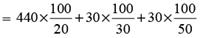= ₹ 2360 cr.